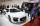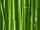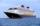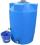Expression of a variable from formula - math word problems - page 20

1. Speed of carThe car went to a city that was 240 km away. If his speed increased by 8 km/h, it would reach the finish one hour earlier. Determine its original speed.
2. BambooBamboo high 32 feet was at a certain height broken by the wind so the bamboo top reached the ground at a distance of 16 feet from the trunk. At what height from the ground was the bamboo broken?
3. Cuboid - VabFind the surface of the cuboid when its volume is 52.8 cubic centimeters, and the length of its two edges is 2 centimeters and 6 centimeters.
4. Finding the baseFind unknown base of percent: 12.5 percent of what = 16 ?
5. A clockA clock was set right at 6:00 AM. If it gains 3 1/2 minutes per hour, what time will it show at 6:00 PM on the same day? Show your solution
6. Three pumpsWe are filling the pool. The first pump would be filled in 12 hours, the second pump in 15 hours. If all three pumps were running at the same time, it would fill the pool for 4 hours. How long would the pool fill only with the third pump?
7. The bulbsThe bulbs are connected serially two bulbs and one resistor. Each bulb has a resistivity of 100 ohms and resistor of 60 ohms The voltage source in the circuit is 24 volts. Do you know what voltage to measure on individual appliances?
8. The cruise shipThe cruise ship has a speed of 12 km / h at a calm surface. When we sail 45 km along the river and 45 km back, it took us exactly 8 hours. Which (constant) speed of flow of the river?
9. MeanA student food the mean of 50 items as 38.6. When checking the work he found that he had taken one item as 50 why it should correctly read as 40 in this circumstances. What should be the correct mean?Given is a regular quadrangular pyramid with a square base. The body height is 30 cm and volume V = 1000 cm³. Calculate its side a and its surface area.
11. Diagonal to areaCalculate the area of a rectangle in which the length of the diagonal is 10 cm.
12. Three pumpsThe water tank is emptied with the first pump in 6 hours, with the second pump in 2 hours. In order to be emptied in 1 hour, all three pumps had to be started at once. How long will take tank be emptied by only the third pump?
13. Cube into sphereThe cube has brushed a sphere as large as possible. Determine how much percent was the waste.
14. The tankThe tank is full up to 4/5 of the total height and contains 240 hl of water. The area of the base is 6 square meters. What is the height of the tank?
15. Minimum surfaceFind the length, breadth, and height of the cuboid shaped box with a minimum surface area, into which 50 cuboid shaped blocks, each with length, breadth and height equal to 4 cm, 3 cm and 2 cm respectively can be packed.
16. EllipseEllipse is expressed by equation 9x2 + 25y2 - 54x - 100y - 44 = 0. Find the length of primary and secondary axes, eccentricity, and coordinates of the center of the ellipse.
17. 3rd dimensionThe block has a surface of 42 dm2 and its dimensions are 3 dm and 2 dm. What is the third dimension?
18. Body diagonalCalculate the volume and surface of the cube if the body diagonal measures 10 dm.
19. The pondWe can see the pond at an angle 65°37'. Its end points are 155 m and 177 m away from the observer. What is the width of the pond?
20. Exponential equationFind x, if 625 ^ x = 5 The equation is exponential because the unknown is in the exponential power of 625

Do you have an interesting mathematical word problem that you can't solve it? Enter it, and we can try to solve it.

To this e-mail address, we will reply solution; solved examples are also published here. Please enter e-mail correctly and check whether you don't have a full mailbox.